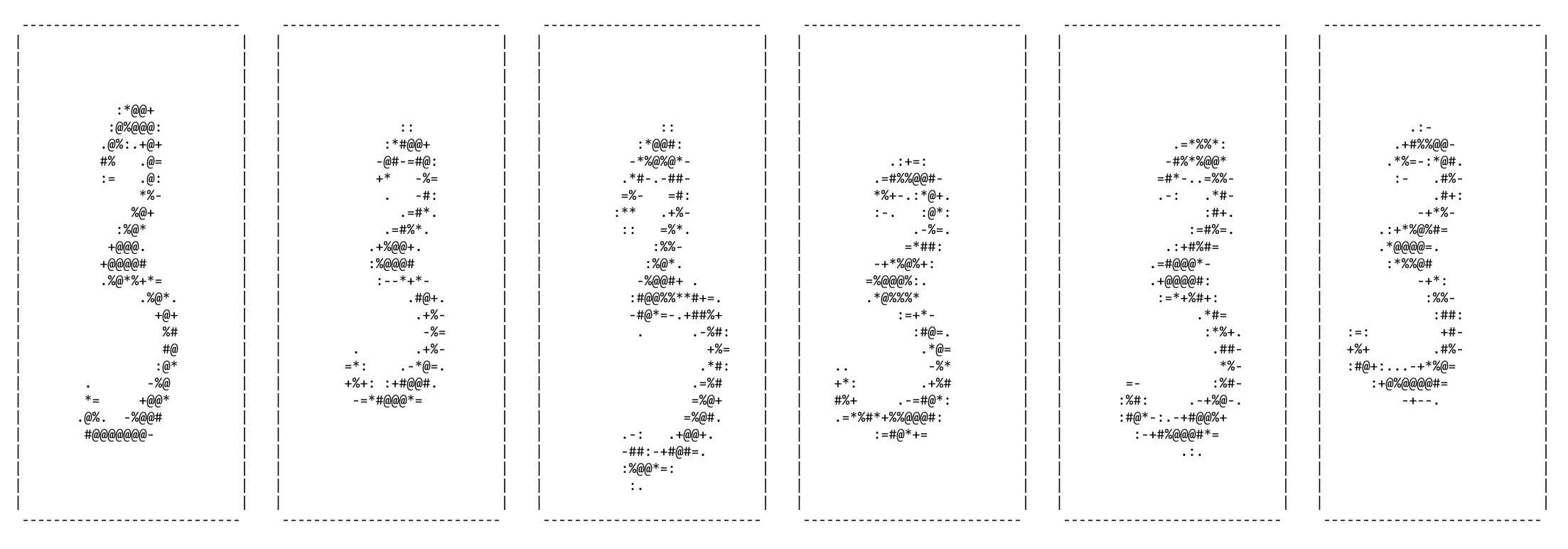It is hard to let go of things you put love into. And yes, I really thought I was done after the 5:th article in this series but then … the accuracy/error metrics from the MNIST example started haunting me and I was quite sure that I could improve on them by implementing another simple trick: Data augmentation. It turned out to be easily implemented and the result was superb. This article is about that.

Also, please check out all parts in this series:

## Data augmentation

Data augmentation is all about fabricating more data from the data you actually got – adding variance without losing the information the data carries. Doing this reduces the risk of overfitting and generally the accuracy on unseen data can be improved.

In the specific case of images as input data (as is the case in the MNIST dataset) augmentation can for instance be:

• Affine transformations (rotation, rescaling, translations etc.)
• Elastic distortions
• Convolutional filters (for instance, and in the MNIST case, making digits thicker or thinner by using max- or min-kernels)

I decided to go for an affine transformation. I’ve used them many times before in CG and know they are very straightforward.

### Affine transformations

Affine transformations map one affine space to another affine space. To be a bit more concrete we can say that an affine transformation can transform a specific coordinate via operations such as rotations, scalings and translations … and tell what that coordinate would be after these changes. The affine transformation can be represented as matrices and can be combined such that a series of transformations still can be expressed as a single matrix.

For instance (and here described in a two-dimension) we could compose a transformation M as this:

$$M = T_{(x, y)}R_{\theta} S_{(x, y)}T_{(-x, -y)}\\$$

… where:

• $$T_{(x, y)}$$ would be a translation along the x- and y-axis of (x, y).
• $$R_{\theta}$$ would be a rotation theta radians.
• $$S_{(x, y)}$$ would be a rescale along the x- and y-axis of (x, y).

When we have this M it is just a matter of multiplying it with input coordinates to get their new location in the target space as defined by M. Conversely we could multiply coordinates from the target space with the inverse of M to go back to the original space.

In Java creating these affine transformation matrices is as simple as this:

This is all we need to transform coordinates in the original MNIST digits to coordinates on new fabricated digits, slightly modified versions of the originals to train the network on.

The method as a whole, which is a mutator on the DigitData-entity (see part 5), looks like this:

As you can see on rows 29-41 the code above also features interpolation which makes the transformed result smoother.

Also there is a check to see whether the resulting digit potentially was transformed in such a way that some part of it spills outside the target 28×28-array. When this seems to be the case we discard that change and try again. If we could not reach a good transformed digit within 5 retries we skip the transformation for this round, falling back on the original digit. This very rarely happens. Next round we might get more lucky and get a valid transformation.

And speaking of rounds. How often do we mutate the input data ? After each epoch of training I transform the entire dataset like this:

This way the neural network never sees the same digit twice (with the rare exception of a few bad-luck transformation attempt as described above). In other words: By data augmentation we have kind of created a dataset that is infinite. Of course this is not true in a strict mathematical meaning … but for all our training purposes the variance of the distribution we picked for our random affine transformations is absolutely sufficient to create a stream of unique data.### The result

The error rate of that small neural network that I had settled on (only 50 hidden neurons, see previous article) was down 1% on average and can now steadily be trained to an error rate in the range 1.7% – 2%.

Small change. Great impact.

Please try out how well one of these trained network performs on a small MNIST Playground I created.

Also, if you want to take a closer look the code is here: https://bitbucket.org/tobias_hill/mnist-example/src/Augmentation/

## One thought on “Extra 1 – Data augmentation”

1.HD says:

Hello,

Thank you for this nice set of articles.
I have run several sets of training and i observed some major differences on the error rate between the runs in my IDE (20% for the firsts epoch) and the runs with an executable JAR (89% for the firsts epoch)
Would you have any idea of the reason of those differences ?